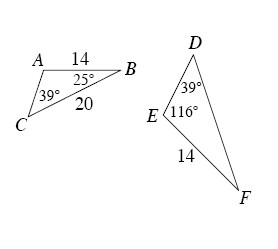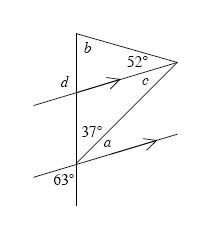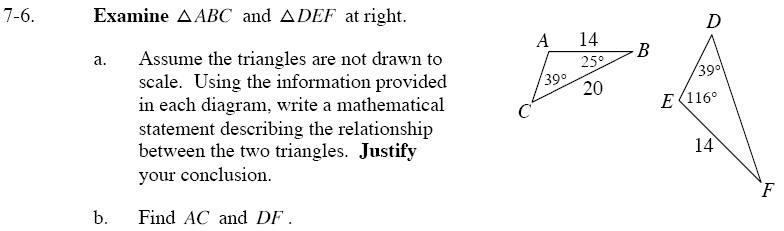### Home > GC > Chapter 7 > Lesson 7.1.1 > Problem7-6

7-6.
1.ΔABC and ΔDEF at right.Homework Help ✎

1. Assume the triangles are not drawn to scale. Using the information provided in each diagram, write a mathematical statement describing the relationship between the two triangles. Justify your conclusion.

2. Find AC and DF.Use the Triangle Angle Sum Theorem to find the unknown angle in each triangle.